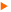Demo CodesHere is a collection of demo programs with increasing complexity to illustrate the main functionalities of the library .

#### 1-D problemsA two-point boundary value problemA 1-D heat equationA 1-D linear transport equation

#### The Laplace equationThe 2-D Laplace equation with P1 finite elementsThe 2-D Laplace equation with P2 finite elementsThe 3-D Laplace equation with P1 finite elementsThe 2-D Steklov-Poincaré problem using P0 boundary elements

#### Heat TransferA 2-D steady state diffusion convection codeA 2-D transient heat transfer codeA 3-D steady state heat transfer code

#### Solid and Structural MechanicsA linear elasticity code with planar deformationsA 3-D linear elasticity codeA linear elasticity code with planar deformations and contactAn elastic beam codeA planar truss code

#### Fluid DynamicsA 2-D incompressible fluid flow problem using quadrilateral finite elementsA 2-D incompressible fluid flow problem using triangular finite elements and a projection method

#### The Linear SolverSolve a linear system by a direct methodSolve a linear system issued from a PDE by a preconditioned iterative method

#### The ODE solverA nonlinear first-order ode given by a regular expressionA first-order nonlinear ode given numericallyA system of first-order linear ode's given numericallyA simple nonlinear ode given by regular expressions

#### The Time Stepping solverSolution of a transient heat transfer problem by the BDF2 schemeSolution of a problem of elastodynamics by the Newmark method

#### The Eigen Problem solverEigenvalues of a given symmetric matrixSolve an eigenvalue problem for the Laplace equation

#### The Optimization solverA one variable problemA mutivariable problemSolution of the Laplace equation as an optimization problem

#### The Nonlinear solverA one variable problem where the function is given by a C-functionA one variable problem where the function is given by a regular expressionSolution of a system of two algebraic equations, the system being defined by regular expressionsSolution of the same system as above, where the functions are given by C-functionsA one variable problem with the objective function given by an algebraic expressionA 2-D mesh adaptation example where the mesh is adapted to a given solution vector